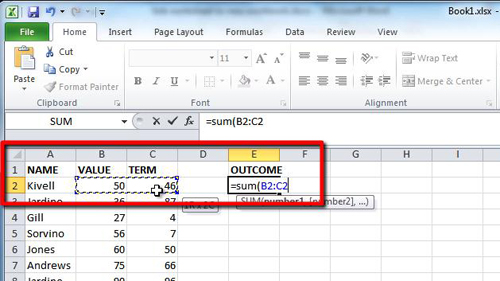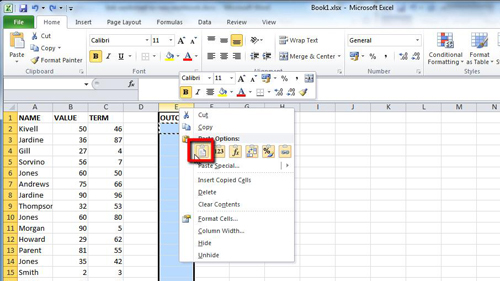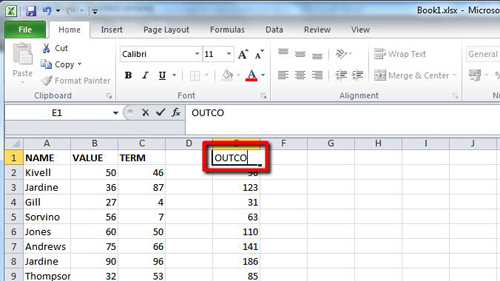## How to Make Excel 2010 formulas for columns

If you have a great deal of data and need to apply many formulas, learning how to make excel formulas for columns is a valuable time saving skill.

Step # 1 – Creating a Template Formula

Start Excel and load the document where you need to apply a formula to the column. Here we want to create a formula in the “OUTCOME” column that will divide the “VALUE” by the “TERM”. Enter “=SUM(“ into cell E2 and then click on cell “B2” and drag to “C2”. Type “)” and press return.Step # 2 – Applying the Formula to the Entire Column

In this case we want to apply this formula to the entire “E” column so that all the values in the “B” and “C” columns are divided like we see with the single formula. Fortunately learning how to make excel formulas for columns is easy as Excel will automatically change the formula to calculate the new values. Copy the contents of cell “E2” and select the entire “E” column. Right click on the column and choose the standard paste option.Step # 3 – Tidying up the Sheet

This will apply the formula to every cell, including the title cell and as you can see here it now reads “0” instead of “OUTCOME”. Click in the cell and type “OUTCOME” again to replace this unwanted “0”.